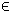## 24SIM Problem

Simulation problems can be of any problem type, but in general they are global black-box problem interfacing an external simulator. The setup may require that objective values and constraints are evaluated simultaneously. To accommodate this in TOMLAB a special assign routine has been developed, simAssign. The solver execution will be much more efficient if using this assign routine.

The simulation problem is identical to the mixed-integer nonlinear programming problem defined as:

 min x
f(x)

s/t
 xL ≤ x ≤ xU bL ≤ A x ≤ bU cL ≤ c(x) ≤ cU
(26)
where x, xL, xURn, f(x)R, ARm1 × n, bL,bURm1 and cL,c(x),cURm2. The variables xI, the index subset of 1,...,n, are restricted to be integers.

Example problem:

The following files define a problem in TOMLAB.

File: tomlab/quickguide/simQG.m, simQG_fc.m, simQG_gdc.m
```  fc:   Function value and nonlinear constraint vector
```
The following file illustrates how to solve this simulation (SIM) problem in TOMLAB. Also view the m-files specified above for more information.

File: tomlab/quickguide/simQG.m

Open the file for viewing, and execute simQG in Matlab.
```% simQG is a small example problem for defining and solving simulation
% problems where the objective function and constraints are evaluated
% during the same function call.

Name = 'HS 47';
b_L  = [];
b_U  = [];
A    = [];
c_L  = [0; 0; 0];
c_U  = [0; 0; 0];
x_0  = [2; sqrt(2); -1; 2-sqrt(2); .5];
x_L  = [];
x_U  = [];

Prob = simAssign('simQG_fc', 'simQG_gdc', [], [], x_L, x_U, ...
Name, x_0, [], A, b_L, b_U, [], c_L, c_U);

Result = tomRun('snopt', Prob, 1);
% Prob.KNITRO.options.HESSOPT = 6;
% Prob.KNITRO.options.ALG = 3;
% Result2 = tomRun('knitro', Prob, 1);```